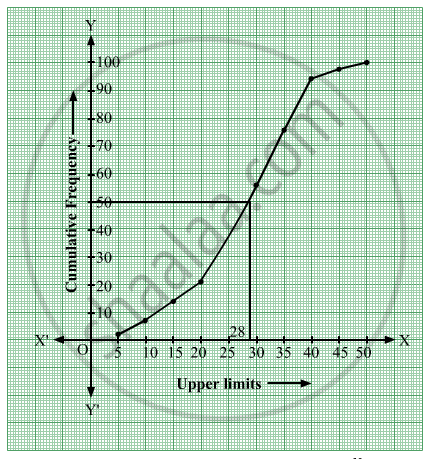# The Marks Obtained by 100 Students of a Class in an Examination Are Given Below.Draw 'A Less Than' Type Cumulative Frequency Curves (Ogive). Hence Find the Median. - Mathematics

Graph
Sum

The marks obtained by 100 students of a class in an examination are given below.

 Mark No. of Student 0 - 5 2 5 - 10 5 10 - 15 6 15 - 20 8 20 - 25 10 25 - 30 25 30 - 35 20 35 - 40 18 40 - 45 4 45 - 50 2

Draw 'a less than' type cumulative frequency curves (ogive). Hence find the median.

#### Solution

We first prepare the cumulative frequency table by less than method as given below–

 Marks No. of students Marks less than Cumulative Frequency 0 - 5 2 5 2 5 - 10 5 10 7 10 - 15 6 15 13 15 - 20 8 20 21 20 - 25 10 25 31 25 - 30 25 30 56 30 - 35 20 35 76 35 - 40 18 40 94 40 - 45 4 45 98 45 - 50 2 50 100

Thus we will plot the points (5, 2), (10, 7), (15, 13), (20, 21), (25, 31), (30, 56), (35, 76), (40, 94), (45, 98) and (50, 100).From the above ogive, the horizontal line drawn from ("N")/(2)=50 intersects the ogive at a point whose x-coordinate is approximately 28.

Hence, Median≈">28.

Concept: Graphical Representation of Cumulative Frequency Distribution
Is there an error in this question or solution?